# Objectives The student will be able to 1

• Slides: 13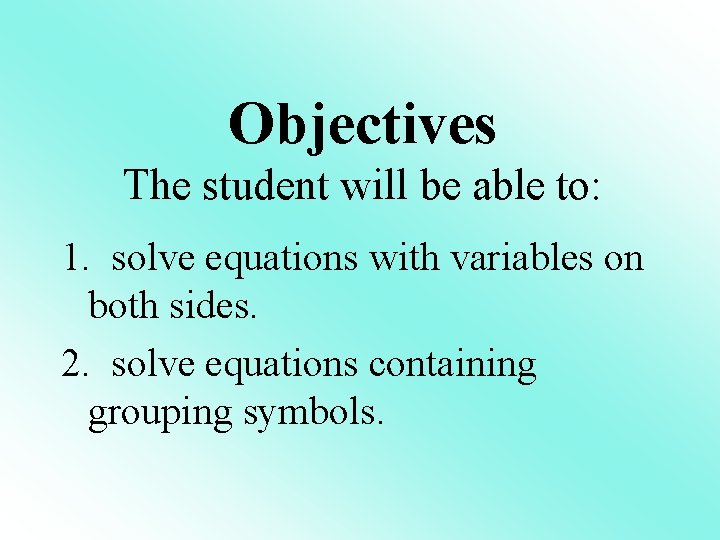Objectives The student will be able to: 1. solve equations with variables on both sides. 2. solve equations containing grouping symbols.1) Solve. 3 x + 2 = 4 x - 1 You need to get the variables on one side of the equation. It does not matter which variable you move. Try to move the one that will keep your variable positive.1. 2. 3. 4. 5. 1) Solve 3 x + 2 = 4 x - 1 Subtract 3 x from - 3 x both sides 2 = x-1 Simplify Add 1 to both +1 +1 sides Simplify 3 = x Check your answer 3(3) + 2 = 4(3) - 1 9 + 2 = 12 - 11. 2. 3. 4. 5. 6. 7. 2) Solve 8 y - 9 = -3 y + 2 + 3 y Add 3 y to both sides 11 y – 9 = 2 Simplify Add 9 to both sides +9 +9 Simplify Divide both sides by 11 11 y = 11 Simplify Check your answer 11 11 y=1 8(1) - 9 = -3(1) + 2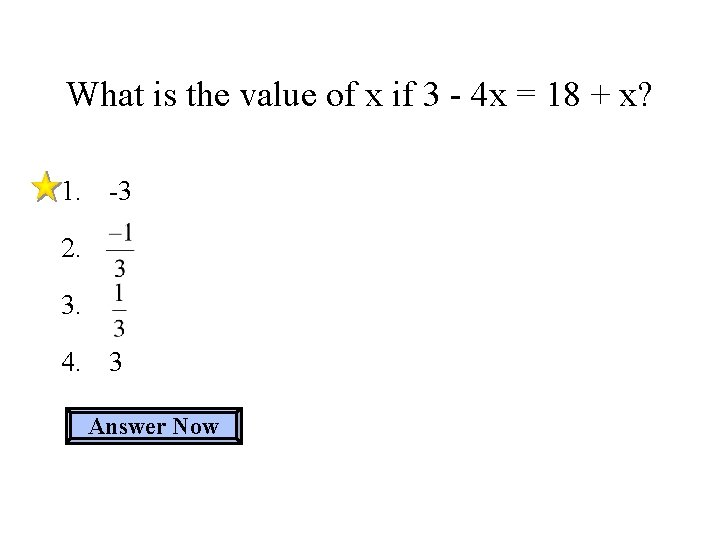What is the value of x if 3 - 4 x = 18 + x? 1. -3 2. 3. 4. 3 Answer Now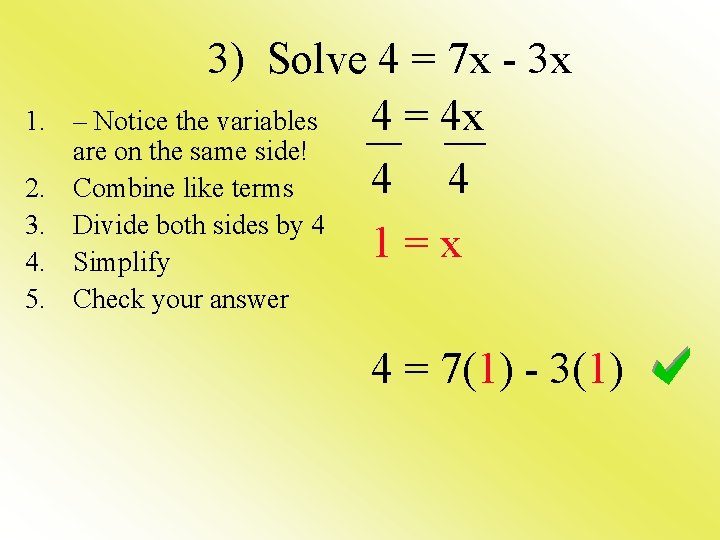1. 3) Solve 4 = 7 x - 3 x – Notice the variables 4 = 4 x are on the same side! 4 4 Combine like terms Divide both sides by 4 1=x Simplify 2. 3. 4. 5. Check your answer 4 = 7(1) - 3(1)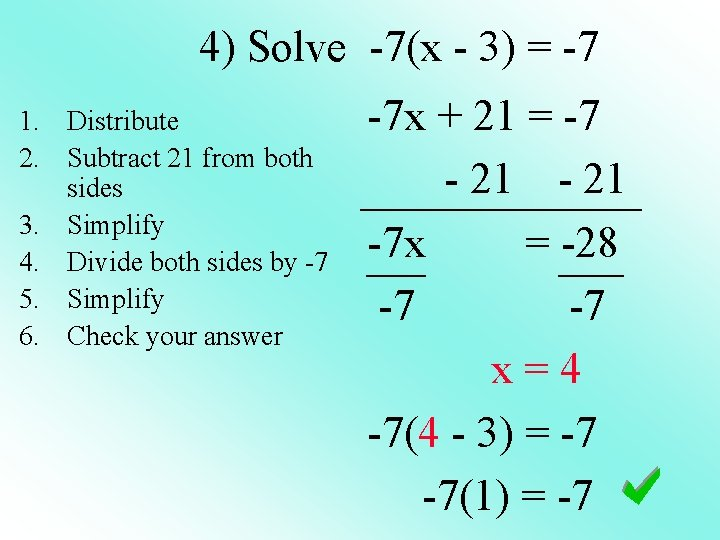4) Solve -7(x - 3) = -7 1. Distribute 2. Subtract 21 from both sides 3. Simplify 4. Divide both sides by -7 5. Simplify 6. Check your answer -7 x + 21 = -7 - 21 -7 x = -28 -7 -7 x=4 -7(4 - 3) = -7 -7(1) = -7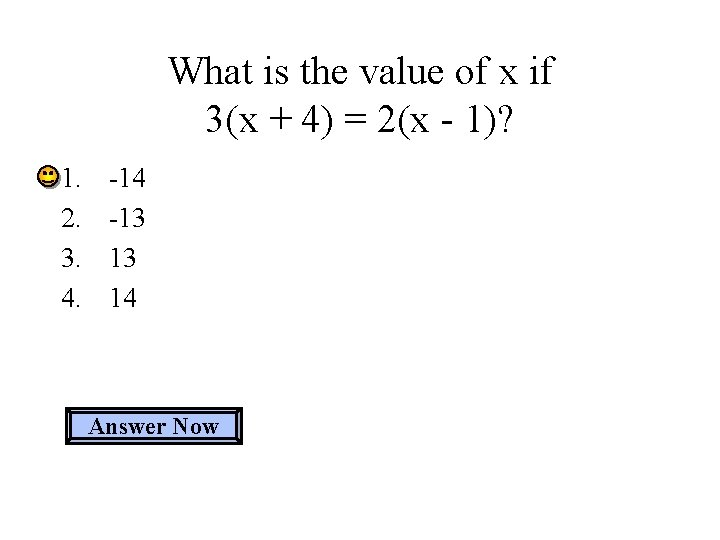What is the value of x if 3(x + 4) = 2(x - 1)? 1. 2. 3. 4. -14 -13 13 14 Answer Now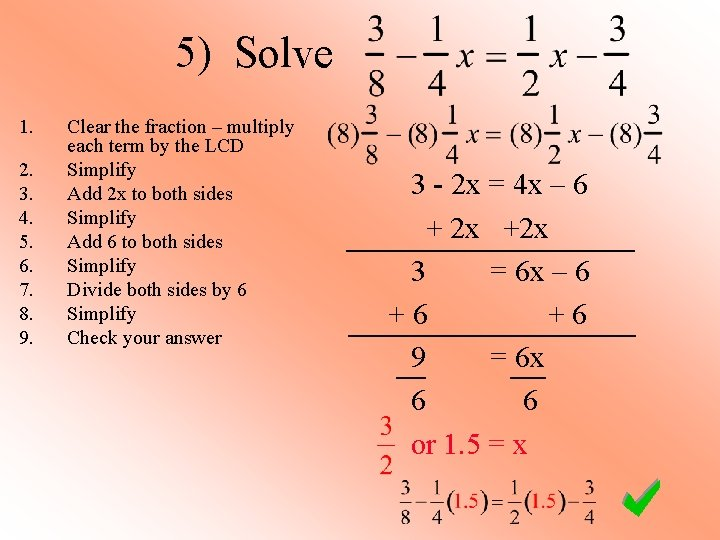5) Solve 1. 2. 3. 4. 5. 6. 7. 8. 9. Clear the fraction – multiply each term by the LCD Simplify Add 2 x to both sides Simplify Add 6 to both sides Simplify Divide both sides by 6 Simplify Check your answer 3 - 2 x = 4 x – 6 + 2 x +2 x 3 = 6 x – 6 +6 +6 9 = 6 x 6 6 or 1. 5 = x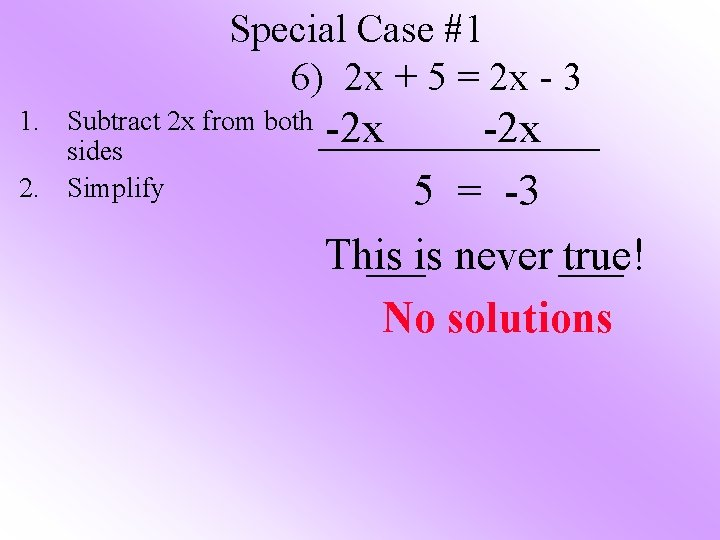Special Case #1 6) 2 x + 5 = 2 x - 3 1. Subtract 2 x from both sides 2. Simplify -2 x 5 = -3 This is never true! No solutions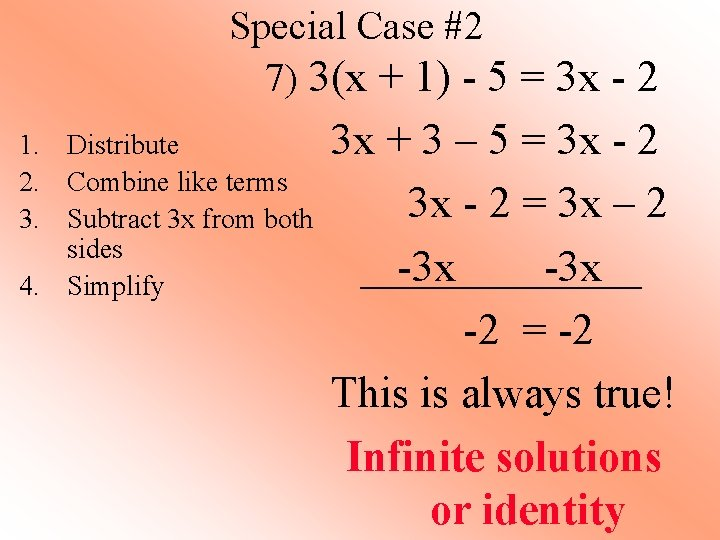Special Case #2 7) 3(x + 1) - 5 = 3 x - 2 1. Distribute 2. Combine like terms 3. Subtract 3 x from both sides 4. Simplify 3 x + 3 – 5 = 3 x - 2 = 3 x – 2 -3 x -2 = -2 This is always true! Infinite solutions or identityWhat is the value of x if -3 + 12 x = 12 x - 3? 1. 2. 3. 4. 0 4 No solutions Infinite solutions Answer Now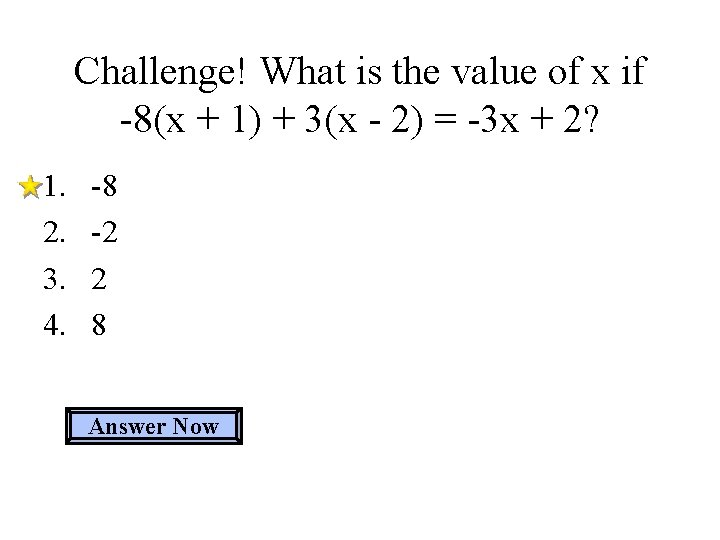Challenge! What is the value of x if -8(x + 1) + 3(x - 2) = -3 x + 2? 1. 2. 3. 4. -8 -2 2 8 Answer Now